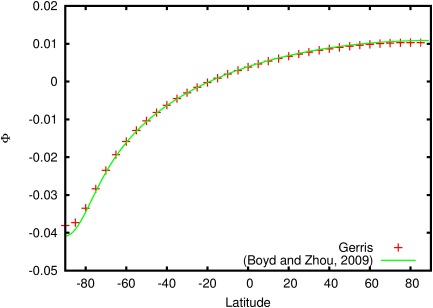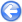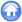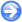### 13.4PASS: Poisson equation on a sphere with Gaussian forcing

Author
Sébastien Delaux
Command
sh gaussian.sh gaussian.gfs 2e-5
Version
110208
Required files
gaussian.sh prof.ref
Running time
6 seconds

This test case is inspired from  who derived an analytical solution for the Poisson equation on a sphere forced by a Gaussian term on the north pole. The equation is of the form

2 Φ = exp(−2 є2 (1−cos(θ+
 π 2
))) − cste

The problem is axisymmetric and has for solution:

Φ =
 1 4є2

1 − exp(−4 є2
log(1−x) −
 1 4є2
exp(−4 є2) log

 1+x 1−x

 1 4є2
E1 (2 є2 (1−x)) +
 1 4є2
exp(−4 є2Ei(2 є2 (1+x))

where E1 is the exponential integral function, Ei(x) = −Re (E1(−x)) and x = cos(θ+π/2).

The solution is not easy to compute and was evaluated over a cross-section using the Maxima software.

 Figure 175: Solution of the Poisson problem as a function of latitude.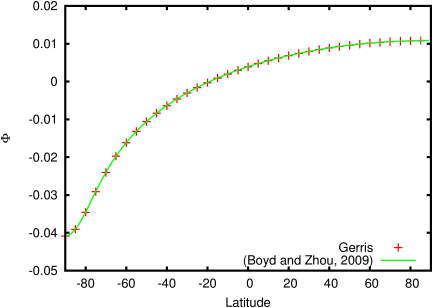#### 13.4.1PASS: Gaussian forcing using longitude-latitude coordinates

Author
Sébastien Delaux
Command
sh ../gaussian.sh lonlat.gfs 4e-3
Version
110208
Required files